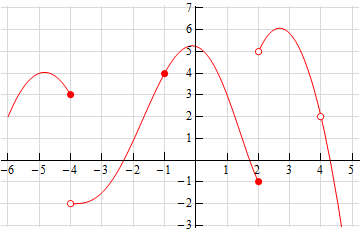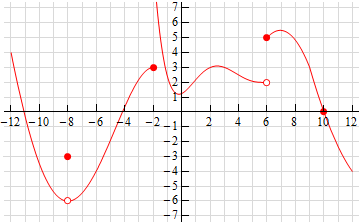Paul's Online Notes
Home / Calculus I / Limits / Continuity
Show Mobile Notice Show All Notes Hide All Notes
Mobile Notice
You appear to be on a device with a "narrow" screen width (i.e. you are probably on a mobile phone). Due to the nature of the mathematics on this site it is best views in landscape mode. If your device is not in landscape mode many of the equations will run off the side of your device (should be able to scroll to see them) and some of the menu items will be cut off due to the narrow screen width.

### Section 2.9 : Continuity

1. The graph of $$f\left( x \right)$$ is given below. Based on this graph determine where the function is discontinuous.Solution
2. The graph of $$f\left( x \right)$$ is given below. Based on this graph determine where the function is discontinuous.Solution

For problems 3 – 7 using only Properties 1 – 9 from the Limit Properties section, one-sided limit properties (if needed) and the definition of continuity determine if the given function is continuous or discontinuous at the indicated points.

1. $$\displaystyle f\left( x \right) = \frac{{4x + 5}}{{9 - 3x}}$$
1. $$x = - 1$$
2. $$x = 0$$
3. $$x = 3$$
Solution
2. $$\displaystyle g\left( z \right) = \frac{6}{{{z^2} - 3z - 10}}$$
1. $$z = - 2$$
2. $$z = 0$$
3. $$z = 5$$
Solution
3. $$g\left( x \right) = \left\{ {\begin{array}{rl}{2x}&{x < 6}\\{x - 1}&{x \ge 6}\end{array}} \right.$$
1. $$x = 4$$
2. $$x = 6$$
Solution
4. $$h\left( t \right) = \left\{ {\begin{array}{rl}{{t^2}}&{t < - 2}\\{t + 6}&{t \ge - 2}\end{array}} \right.$$
1. $$t = - 2$$
2. $$t = 10$$
Solution
5. $$g\left( x \right) = \left\{ {\begin{array}{rc}{1 - 3x}&{x < - 6}\\7&{x = - 6}\\{{x^3}}&{ - 6 < x < 1}\\1&{x = 1}\\{2 - x}&{x > 1}\end{array}} \right.$$
1. $$x = - 6$$
2. $$x = 1$$
Solution

For problems 8 – 12 determine where the given function is discontinuous.

1. $$\displaystyle f\left( x \right) = \frac{{{x^2} - 9}}{{3{x^2} + 2x - 8}}$$ Solution
2. $$\displaystyle R\left( t \right) = \frac{{8t}}{{{t^2} - 9t - 1}}$$ Solution
3. $$\displaystyle h\left( z \right) = \frac{1}{{2 - 4\cos \left( {3z} \right)}}$$ Solution
4. $$\displaystyle y\left( x \right) = \frac{x}{{7 - {{\bf{e}}^{2x + 3}}}}$$ Solution
5. $$g\left( x \right) = \tan \left( {2x} \right)$$ Solution

For problems 13 – 15 use the Intermediate Value Theorem to show that the given equation has at least one solution in the indicated interval. Note that you are NOT asked to find the solution only show that at least one must exist in the indicated interval.

1. $$25 - 8{x^2} - {x^3} = 0$$ on $$\left[ { - 2,4} \right]$$ Solution
2. $${w^2} - 4\ln \left( {5w + 2} \right) = 0$$ on $$\left[ {0,4} \right]$$ Solution
3. $$4t + 10{{\bf{e}}^t} - {{\bf{e}}^{2t}} = 0$$ on $$\left[ {1,3} \right]$$ Solution